# module mlmodel.decision_tree_logreg#

## Short summary#

module mlinsights.mlmodel.decision_tree_logreg

Builds a tree of logistic regressions.

source on GitHub

## Classes#

class

truncated documentation

_DecisionTreeLogisticRegressionNode

Describes the tree structure hold by class DecisionTreeLogisticRegression. See also notebook Decision Tree and Logistic Regression. …

DecisionTreeLogisticRegression

Fits a logistic regression, then fits two other logistic regression for every observation on both sides of the border. …

## Functions#

function

truncated documentation

likelihood

Computes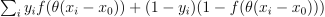where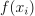is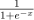. …

logistic

Computes.

## Properties#

property

truncated documentation

_repr_html_

HTML representation of estimator. This is redundant with the logic of _repr_mimebundle_. The latter should …

tree_depth_

Returns the maximum depth of the tree.

tree_depth_

Returns the maximum depth of the tree.

## Methods#

method

truncated documentation

__init__

constructor

__init__

constructor

_fit_parallel

Implements the parallel strategy.

_fit_perpendicular

Implements the perpendicular strategy.

decision_function

Calls decision_function.

decision_path

Returns the decision path.

decision_path

Returns the classification probabilities.

enumerate_leaves_index

Returns the leaves index.

fit

Builds the tree model.

fit

Fits a logistic regression, then splits the sample into positive and negative examples, finally tries to fit …

fit_improve

The method only works on a linear classifier, it changes the intercept in order to be within the constraints …

get_leaves_index

Returns the index of every leave.

predict

Runs the predictions.

predict

Predicts

predict_proba

Converts predictions into probabilities.

predict_proba

Returns the classification probabilities.

## Documentation#

Builds a tree of logistic regressions.

source on GitHub

class mlinsights.mlmodel.decision_tree_logreg.DecisionTreeLogisticRegression(estimator=None, max_depth=20, min_samples_split=2, min_samples_leaf=2, min_weight_fraction_leaf=0.0, fit_improve_algo='auto', p1p2=0.09, gamma=1.0, verbose=0, strategy='parallel')#

Fits a logistic regression, then fits two other logistic regression for every observation on both sides of the border. It goes one until a tree is built. It only handles a binary classification. The built tree cannot be deeper than the maximum recursion.

Parameters:
• estimator – binary classification estimator, if empty, use a logistic regression, the theoritical model defined with a logistic regression but it could any binary classifier

• max_depth – int, default=None The maximum depth of the tree. If None, then nodes are expanded until all leaves are pure or until all leaves contain less than min_samples_split samples. It must be below the maximum allowed recursion by python.

• min_samples_split

int or float, default=2 The minimum number of samples required to split an internal node: - If int, then consider min_samples_split as the minimum number. - If float, then min_samples_split is a fraction and

ceil(min_samples_split * n_samples) are the minimum number of samples for each split.

• min_samples_leaf

int or float, default=1 The minimum number of samples required to be at a leaf node. A split point at any depth will only be considered if it leaves at least min_samples_leaf training samples in each of the left and right branches. This may have the effect of smoothing the model, especially in regression. - If int, then consider min_samples_leaf as the minimum number. - If float, then min_samples_leaf is a fraction and

ceil(min_samples_leaf * n_samples) are the minimum number of samples for each node.

• min_weight_fraction_leaf – float, default=0.0 The minimum weighted fraction of the sum total of weights (of all the input samples) required to be at a leaf node. Samples have equal weight when sample_weight is not provided.

• fit_improve_algo

string, one of the following value: - ‘auto’: chooses the best option below, ‘none’ for

every non linear model, ‘intercept_sort’ for linear models

• none’: does not nothing once the binary classifier is fit

• ’intercept_sort’: if one side of the classifier is too small, the method changes the best intercept possible verifying the constraints

• ’intercept_sort_always’: always chooses the best intercept possible

• p1p2 – threshold in [0, 1] for every split, we can define probabilities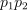which define the ratio of samples in both splits, ifis below the threshold, method fit_improve is called

• gamma – weight before the coefficient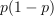. When the model tries to improve the linear classifier, it looks a better intercept which maximizes the likelihood and verifies the constraints. In order to force the classifier to choose a value which splits the dataset into 2 almost equal folds, the function maximimes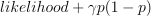where p is the proportion of samples falling in the first fold.

• verbose – prints out information about the training

• strategy‘parallel’ or ‘perpendicular’, see below

Fitted attributes:

• classes_: ndarray of shape (n_classes,) or list of ndarray

The classes labels (single output problem), or a list of arrays of class labels (multi-output problem).

• tree_: Tree

The underlying Tree object.

The class implements two strategies to build the tree. The first one ‘parallel’ splits the feature space using the hyperplan defined by a logistic regression, the second strategy ‘perpendicular’ splis the feature space based on a hyperplan perpendicular to a logistic regression. By doing this, two logistic regression fit on both sub parts must necessary decreases the training error.

source on GitHub

constructor

__init__(estimator=None, max_depth=20, min_samples_split=2, min_samples_leaf=2, min_weight_fraction_leaf=0.0, fit_improve_algo='auto', p1p2=0.09, gamma=1.0, verbose=0, strategy='parallel')#

constructor

_fit_improve_algo_values = (None, 'none', 'auto', 'intercept_sort', 'intercept_sort_always')#
_fit_parallel(X, y, sample_weight)#

Implements the parallel strategy.

_fit_perpendicular(X, y, sample_weight)#

Implements the perpendicular strategy.

decision_function(X)#

Calls decision_function.

source on GitHub

decision_path(X, check_input=True)#

Returns the decision path.

Parameters:
• X – inputs

• check_input – unused

Returns:

sparse matrix

source on GitHub

fit(X, y, sample_weight=None)#

Builds the tree model.

Parameters:
• X – numpy array or sparse matrix of shape [n_samples,n_features] Training data

• y – numpy array of shape [n_samples, n_targets] Target values. Will be cast to X’s dtype if necessary

• sample_weight – numpy array of shape [n_samples] Individual weights for each sample

Returns:

self : returns an instance of self.

Fitted attributes:

source on GitHub

get_leaves_index()#

Returns the index of every leave.

source on GitHub

predict(X)#

Runs the predictions.

source on GitHub

predict_proba(X)#

Converts predictions into probabilities.

source on GitHub

property tree_depth_#

Returns the maximum depth of the tree.

source on GitHub

class mlinsights.mlmodel.decision_tree_logreg._DecisionTreeLogisticRegressionNode(estimator, threshold=0.5, depth=1, index=0)#

Bases: object

Describes the tree structure hold by class DecisionTreeLogisticRegression. See also notebook Decision Tree and Logistic Regression.

source on GitHub

constructor

Parameters:

estimator – binary estimator

source on GitHub

__init__(estimator, threshold=0.5, depth=1, index=0)#

constructor

Parameters:

estimator – binary estimator

source on GitHub

decision_path(X, mat, indices)#

Returns the classification probabilities.

Parameters:
• X – features

• mat – decision path (allocated matrix)

source on GitHub

enumerate_leaves_index()#

Returns the leaves index.

source on GitHub

fit(X, y, sample_weight, dtlr, total_N)#

Fits a logistic regression, then splits the sample into positive and negative examples, finally tries to fit logistic regressions on both subsamples. This method only works on a linear classifier.

Parameters:
• X – features

• y – binary labels

• sample_weight – weights of every sample

• total_N – total number of observation

Returns:

last index

source on GitHub

fit_improve(dtlr, total_N, X, y, sample_weight)#

The method only works on a linear classifier, it changes the intercept in order to be within the constraints imposed by the min_samples_leaf and min_weight_fraction_leaf. The algorithm has a significant cost as it sorts every observation and chooses the best intercept.

Parameters:
• total_N – total number of observations

• X – features

• y – labels

• sample_weight – sample weight

Returns:

probabilities

source on GitHub

predict(X)#

Predicts

source on GitHub

predict_proba(X)#

Returns the classification probabilities.

Parameters:

X – features

Returns:

probabilties

source on GitHub

property tree_depth_#

Returns the maximum depth of the tree.

source on GitHub

mlinsights.mlmodel.decision_tree_logreg.likelihood(x, y, theta=1.0, th=0.0)#

Computeswhereis.

source on GitHub

mlinsights.mlmodel.decision_tree_logreg.logistic(x)#

Computes.

source on GitHub